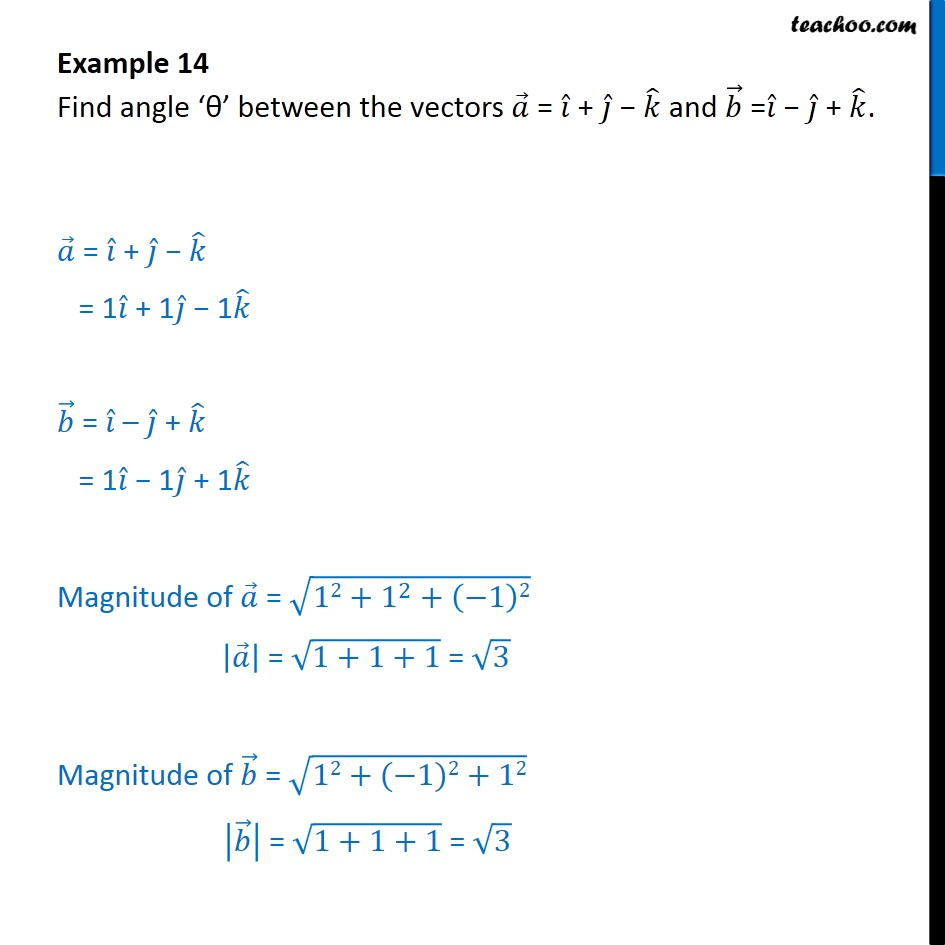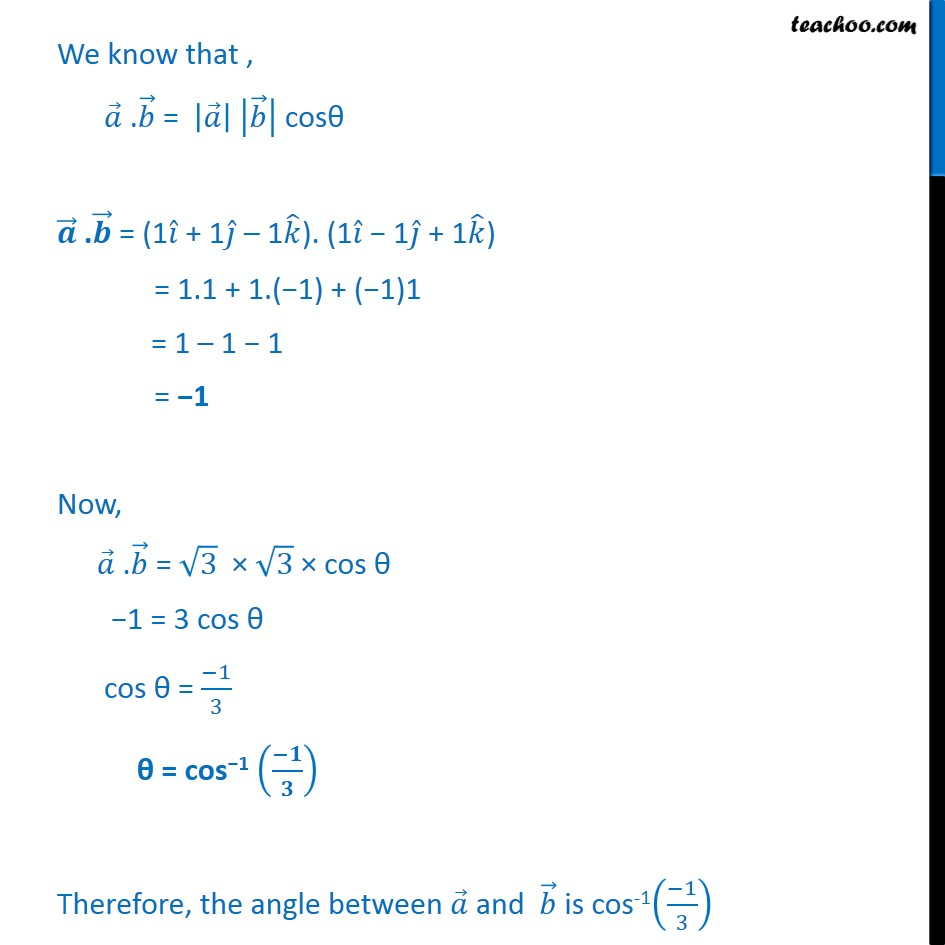1. Class 12
2. Important Question for exams Class 12
3. Chapter 10 Class 12 Vector Algebra

Transcript

Example 14 Find angle ‘θ’ between the vectors 𝑎﷯ = 𝑖﷯ + 𝑗﷯ − 𝑘﷯ and 𝑏﷯ = 𝑖﷯ − 𝑗﷯ + 𝑘﷯. 𝑎﷯ = 𝑖﷯ + 𝑗﷯ − 𝑘﷯ = 1 𝑖﷯ + 1 𝑗﷯ − 1 𝑘﷯ 𝑏﷯ = 𝑖﷯ – 𝑗﷯ + 𝑘﷯ = 1 𝑖﷯ − 1 𝑗﷯ + 1 𝑘﷯ Magnitude of 𝑎﷯ = ﷮12+ 1﷮2﷯+ −1﷯2﷯ 𝑎﷯﷯ = ﷮1+1+1﷯ = ﷮3﷯ Magnitude of 𝑏﷯ = ﷮12+(−1)2+12﷯ 𝑏﷯﷯ = ﷮1+1+1﷯ = ﷮3﷯ We know that , 𝑎﷯ . 𝑏﷯ = 𝑎﷯﷯ 𝑏﷯﷯ cosθ 𝒂﷯ . 𝒃﷯ = (1 𝑖﷯ + 1 𝑗﷯ – 1 𝑘﷯). (1 𝑖﷯ − 1 𝑗﷯ + 1 𝑘﷯) = 1.1 + 1.(−1) + (−1)1 = 1 – 1 − 1 = −1 Now, 𝑎﷯ . 𝑏﷯ = ﷮3﷯ × ﷮3﷯ × cos θ −1 = 3 cos θ cos θ = −1﷮3﷯ θ = cos−1 −𝟏﷮𝟑﷯﷯ Therefore, the angle between 𝑎﷯ and 𝑏﷯ is cos-1 −1﷮3﷯﷯

Chapter 10 Class 12 Vector Algebra

Class 12
Important Question for exams Class 12